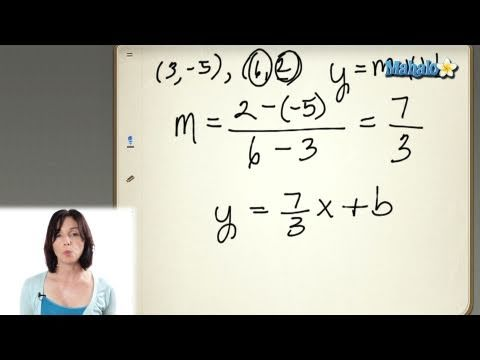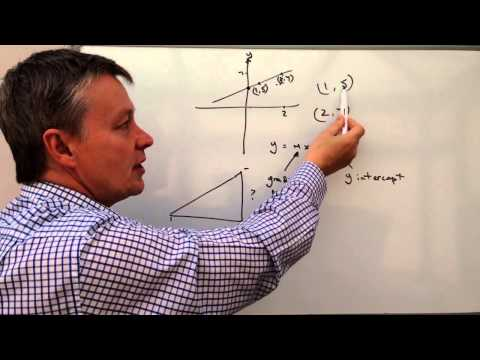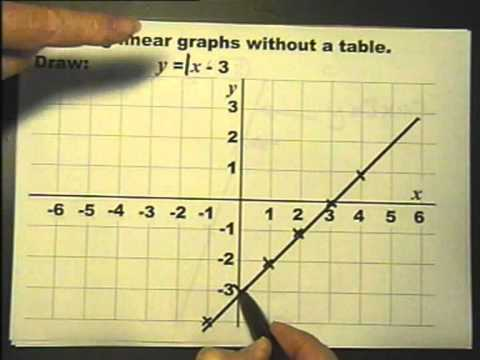## Three Standard form of Equation of Straight line

Subject: Optional Mathematics

#### Overview

This note contents various method or way to measure the slope intercept.

#### Slope intercept form

To find the equation of a straight line in form of y = mx + c
Let a straight line XY make an intercept c on Y-axis. then OY = c.
Let m be the slope of the line and $\theta$ be its inclination.
Then, m = tanΘ
Let P(x, y) be any point on the XY. Draw perpendicular TR from P to X-axis.
Then, OR = x and RT = y.
Again, draw perpendicular YS from Y to the line segment TR, then
YS = OR = x
ST = RT - RS = RT - OY = y - c
Also,
∠TYS = ∠YXO = $\theta$
From right angled ΔYTS,
tanΘ = $\frac{ST}{YS}$
or, m = $\frac{y - c}{x}$
or, y - c = mx
or, y = mx + c, which is the equation of a straight line in the required form.

#### Double intercept form

To find the equation of a straight line in the form $\frac{x}{a}$ + $\frac{y}{b}$ = 1
Let the straight line EF cut the axis at E and F. Let OE = a and OF = b. These are the intercept on the X-axis and the Y-axis respectively. Obviously, the coordinates of E and F are respectively (a, 0) and (0, b).
Let G(x, y) be any point on the line EF. Then,
Slope of the line segment EG = $\frac{y - 0}{x - a}$
Slope of the line segment GF= $\frac{b -y}{0 - x}$
But EG and GF are the segments of the same straight line.
So, $\frac{y - 0}{x - a}$ = $\frac{b - y}{0 - x}$
or, -xy = bx - ab - xy + ay
Dividing both sides by ab we get
$\frac{x}{a}$ + $\frac{y}{b}$ = 1, which bis the equation of straight line in the required form.

#### Normal form or Perpendicular form

To find the equation of a straight line in the form x cos∝ + y sin∝ = p
Let a straight line XY cut the axes at X and Y. Then,
X-intercept = OX and Y-intercept =OY
Then, equation of the line XY is given by
$\frac{x}{OX}$ + $\frac{y}{OY}$ = 1 . . . . . . . . . . . . . . . . . (i)
Draw perpendicular OZ from origin to the line XY.
Let OZ = p and∠XOZ = $\alpha$.
Then, ∠YOZ = 90° - $\alpha$ and
∠OYZ =90° - ∠YOZ = 90° - (90° - ∝) = ∝
From right angled triangle OXZ,
sec∝ = $\frac{OX}{OZ}$
∴ OX = OZ sec$\alpha$ = p sec$\alpha$
From right angled triangle OYZ,
cosec∝ = $\frac{OY}{OZ}$
∴ OY = OZ cosec$\alpha$ = p cosec$\alpha$
Now,
putting the values of OA and OB in (i) we get.
$\frac{x}{p \: secα} + \frac{y}{p \: cosecα}$ = 1
or, $\frac{x \: cosα}{p} + \frac{y \: sinα}{p}$ = 1
or, x cosα + y sinα = p, which is the equation of a straight line in the required form.

##### Things to remember
• y = mx+ c
• $\frac{x}{a}$ + $\frac{y}{b}$ = 1
• x cos∝ + y sin∝ = p
• It includes every relationship which established among the people.
• There can be more than one community in a society. Community smaller than society.
• It is a network of social relationships which cannot see or touched.
• common interests and common objectives are not necessary for society.
##### Videos for Three Standard form of Equation of Straight line##### Finding the Equation of a Line in Slope-Intercept Form##### How to find the equation of a straight line graph with two points##### The general equation of a straight line.

Solution:

Here,
Inclination of the line (θ) = 45°
∴ Slope of the line (m) = tanθ
= tan 45°
= 1
y-intercept (c) = 2
∴ Equation of the line in slope intercept form is given by, y = mx + c
So,
y = 1 . x + 2
or, y - x - 2 = 0 is the required equation.

Solution:

Here,
Inclination of the line (θ) = 60°
∴ Slope of the line (m) = tan 60° = $\sqrt{3}$
∴ Equation of the line is given by, y = mx + c
or, y = $\sqrt{3}$ x + c . . . . . . . . . . . . (1)
Since the line passes though (0, 5)
5 = c
∴ c = 5
Putting the value of c in   (1)
y = $\sqrt{3}$ x + 5 is the required equation.

Here,
X-intercept (a) = 3
Y-intercept (b) = -4
Equation of a staright line is given by $\frac{x}{a}$ + $\frac{y}{b}$ = 1
or, $\frac{x}{3}$ - $\frac{y}{4}$ = 1
or, 4x - 3y = 12
4x - 3y - 12 = 0 is the required equation.

Solution:

Here,
The line cuts off, equal; intercepts on the axes equal in magnitude but opposite in sign.
So, X-intercept = -Y-intercept, i.e. a = -b.
So, its equation is given by $\frac{x}{a}$ + $\frac{y}{b}$ = 1
or, x - y = a . . . . . . . . . (i)
Since it passes through the point (6, 5)
or, 6 - 5 = a
∴ a =  1
Putting the value of a in equation (i)
x - y - 1 = 0 is the required equation.

Solution:

Let AB be the required line that cuts X-axis at A and Y-axis at B such that OA = a and OB = b.
By the questions, C(-2, 3) divides the line AB in the ratio 3 : 4.
Using section formula
or, x = $\frac{m_1x_2 + m_2x_1}{m_1 + m_2}$, y =  $\frac{m_1y_2 + m_2y_1}{m_1 + m_2}$
or, -2 = $\frac{3.0 + 4.a}{3 + 4}$, 3 = $\frac{3.b + 4.0}{3 + 4}$
∴ a = $\frac{-7}{2}$, ∴ b = 7
The equation of line is $\frac{x}{a}$ + $\frac{y}{b}$ = 1
$\frac{x}{\frac{7}{-2}}$ + $\frac{y}{7}$ = 1
∴ 2x - y + 7 = 0 is the required equation.

Solution:

Here,
perpendicular length (p) = 3
Angle made by perpendicular in the positive direction with X-axis (α) = 120°
Equation of the line is given by x cosα + y sinα = p
or, x . cos 120° + y sin 120° = 3
or, x . ($\frac{-1}{2}$) + y . $\frac{\sqrt{3}}{2}$ = 3
or, -x + $\sqrt{3}$ y = 6
∴ x - $\sqrt{3}$ y + 6 = 0 is the required equation.